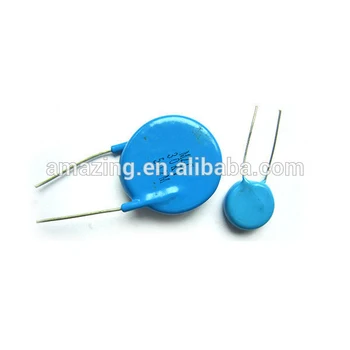## January 7th, 2020

### Емкость конденсатора из двух круглых пластин: точное решение

Красивая статья, показывающая, насколько это сложная задача, если пытаться решить ее не в первом приближении. Море суровой матфизики в наличии, наслаждайтесь:)

Analytical results for the capacitance of a circular plate capacitor: https://arxiv.org/abs/2001.01142

We study the classic problem of the capacitance of a circular parallel plate capacitor. At small separations between the plates, it is initially considered in 19th century by Kirchhoff who found the leading and the subleading term in the capacitance. Despite a large interest in the problem, one and a half century later, analytically was found only the second subleading term. Using the recent advances in the asymptotic analysis of Fredholm integral equations of the second kind with finite support, here we study the one governing the circular capacitor, known as the Love equation. We found analytically many new subleading terms in the capacitance at small separations. We also calculated the asymptotic expansion at large separations, thus providing the two simple expressions which practically describe the capacitance at all distances. The approach described here could be used to find exact analytical expansions for the capacitance to an arbitrary number of terms in both regimes of small and large separations.

Картинка для привлечения внимания:### Лягушечка

Размер малышки можно оценить, сравнив с размером песчинок:)